CSE 5311 Section 501 Spring 1998
Homework 2
Due: February 16, 1998, in class

1. Recall that a sorting algorithm is stable if the original ordering for duplicate keys is preserved. Which of the following sorting algorithms are stable, and which are not? Describe a method for making any sorting algorithm stable.

• Insertion sort - stable. When the key is inserted into the sorted subsequence, only elements with value less than key are moved over to make room for the key.
• Merge Sort - stable. When the Merge function compares the next item in both subsequences, the item from the first (leftmost) subsequence is copied first if the values are equal.
• Heapsort - not stable. If the left and right child of a node have equal value (both greater than the node value) , the left child will be picked to swap with the parent. Consider the sequence 2, 3, 5, 3, 1. The order of the 3s will be reversed.
• Quicksort - not stable. In general the stability depends on the partition algorithm.
• Counting Sort - stable. This is shown in class.

Any unstable sorting algorithm can be made stable by adding an extra field to the sequence entries. Instead of sorting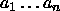, sort. When comparing two items,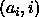is considered to be <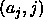iff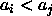or bothand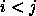.

2. The Quicksearch Center is hired to design a data structure for storing 20,000 names. The client informs the Center that one-fifth of the names will account for four-fifths of the successful searches, and the remaining four-fifths of the names will account for only one-fifth of the successful searches. (There will also be searches for names not in the data structure at all.) The Center first decides to store all 20,000 names in an open-addressed hash table of size 24,000 using double hashing. Then one of its employees, C. Wizard, suggests splitting the 24,000 locations into two tables, a small table of size 6000 to hold the high-frequency fifth of the names (and to be searched first) and a larger table of size 18,000 to hold the low-frequency four-fifths.

1. Is Wizard's suggestion a good one? Analyze both proposals with respect to their performance in the case of both successful and unsuccessful searches.

Idea 1

The load factor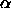here is n/m = 20,000/24,000 = 0.83. Thus the number of probes in an unsuccessful search is, and the expected number of probes in a successful search is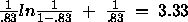.

Idea 2

The load factor for the high-frequency database is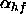= 4,000/6,000 = .67, and the load factor for the low-frequency database is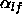= 16,000/18,000 = .89.

An unsuccessful search will first look through hf then lf, and the expected number of probes is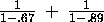= 3.03 + 9.09 = 12.12.

The successful search has two cases: either the key is found in hf which is searched first, or hf is searched first then the key is found in lf. In the first case, the number of expected probes is equal to a successful search in hf, or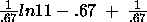= 1.65 + 1.49 = 3.14. In the second case, the number of expected probes is equal to the number of probes for unsuccessful search in hf plus the number of expected probes for a successful search in lf, or 3.03 +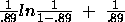= 3.03 + 3.59 = 6.62.

The total number of expected probes for a successful search is weighted by the probability of finding the probe in case 1 or case 2. Thus the total number of expected probes is 0.8*3.14 + 0.2*6.62 = 3.84. Thus idea 1 performs better for unsuccessful and successful searches than idea 2.

2. Suppose that the proportions in the statement of the problem are not 1/5 and 4/5 but p and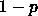, where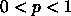. For what values of p, if any, would it make sense to isolate the fraction p of the most frequently occurring keys in a subtable consisting of the fraction p of the available memory?

For idea 1 the values will always be the same (5.88 probes for an unsuccessful search, 3.33 probes for a successful search). Idea 2 will perform better in the case of a successful search when. The value of p will need to be smaller than 1/5 given the same load factors.

3. Show the red-black trees that result after successively inserting the keys 1, 2, 3, 5, 7, 6, 4, 8, 10, 9 into an initially empty red-black tree. Show each insert case as it is applied.

```Inserting 1...
1(B)

Inserting 2...
2(R)
1(B)

Inserting 3...
Insert Case IIIb, x =  3(R)
3(R)
2(B)
1(R)

Insert Case Ib, x =  5(R)
5(R)
3(B)
2(B)
1(B)

Inserting 7...
Insert Case IIIb, x =  7(R)
7(R)
5(B)
3(R)
2(B)
1(B)

Inserting 6...
Insert Case Ib, x =  6(R)
7(B)
6(R)
5(R)
3(B)
2(B)
1(B)

Inserting 4...
7(B)
6(R)
5(R)
4(R)
3(B)
2(B)
1(B)

Inserting 8...
8(R)
7(B)
6(R)
5(R)
4(R)
3(B)
2(B)
1(B)
Inserting 10...
Insert Case Ib, x = 10(R)
Insert Case IIIb, x =  7(R)
10(R)
8(B)
7(R)
6(B)
5(B)
4(R)
3(B)
2(R)
1(B)
Inserting 9...
Insert Case IIb x =  9(R)
Insert Case IIIb, x = 10(R)
10(R)
9(B)
8(R)
7(R)
6(B)
5(B)
4(R)
3(B)
2(R)
1(B)```
4. Starting with the tree at the end of the previous problem, show the red-black trees that result from the successive deletion of the keys in the order 4, 3, 1, 2, 9, 10, 7, 6, 5, 8 Show each delete case as it is applied.

```Deleting 4...
10(R)
9(B)
8(R)
7(R)
6(B)
5(B)
3(B)
2(R)
1(B)

Deleting 3...
Delete Case IIb, x = NIL(B)
10(R)
9(B)
8(R)
7(R)
6(B)
5(B)
2(B)
1(R)

Deleting 1...
10(R)
9(B)
8(R)
7(R)
6(B)
5(B)
2(B)

Deleting 2...
Delete Case Ia, x = NIL(B)
Delete Case IIa, x = NIL(B)
10(R)
9(B)
8(R)
7(B)
6(R)
5(B)

Deleting 9...
10(B)
8(R)
7(B)
6(R)
5(B)

Deleting 10...
8(B)
7(B)
6(R)
5(B)

Deleting 7...
Delete Case IIIb, x = NIL(B)
Delete Case IVb, x = NIL(B)
8(B)
6(B)
5(B)

Deleting 6...
Delete Case IIb, x = NIL(B)
8(B)
5(R)

Deleting 5...
8(B)

Deleting 8...
NIL(B)```